# RD Sharma Solutions for Class 8 Maths Chapter 6 - Algebraic Expressions and Identities Exercise 6.5

To excel in board exams, students can access free pdf of RD Sharma Solutions for Class 8 Maths Exercise 6.5 of Chapter 6 Algebraic Expressions and Identities which are provided here. BYJU’S experts have designed solutions to help students secure good marks in their exams by practising the RD Sharma Solutions thoroughly. The pdf’s can be downloaded easily from the links given below. In Exercise 6.5 of Chapter 6 algebraic expressions and identities, we shall discuss problems based on the multiplication of two binomials.

## Download the pdf of RD Sharma Solutions For Class 8 Maths Exercise 6.5 Chapter 6 Algebraic Expressions and Identities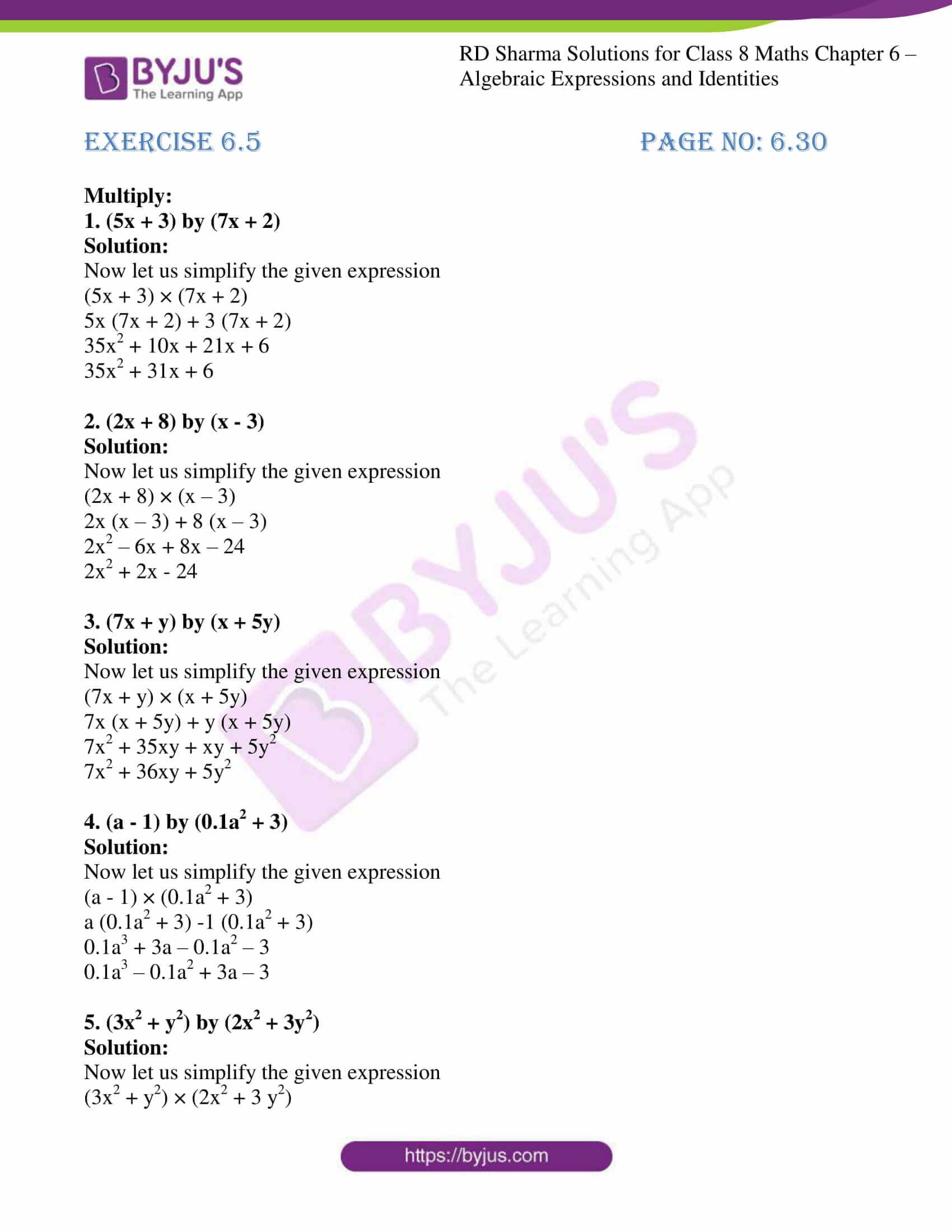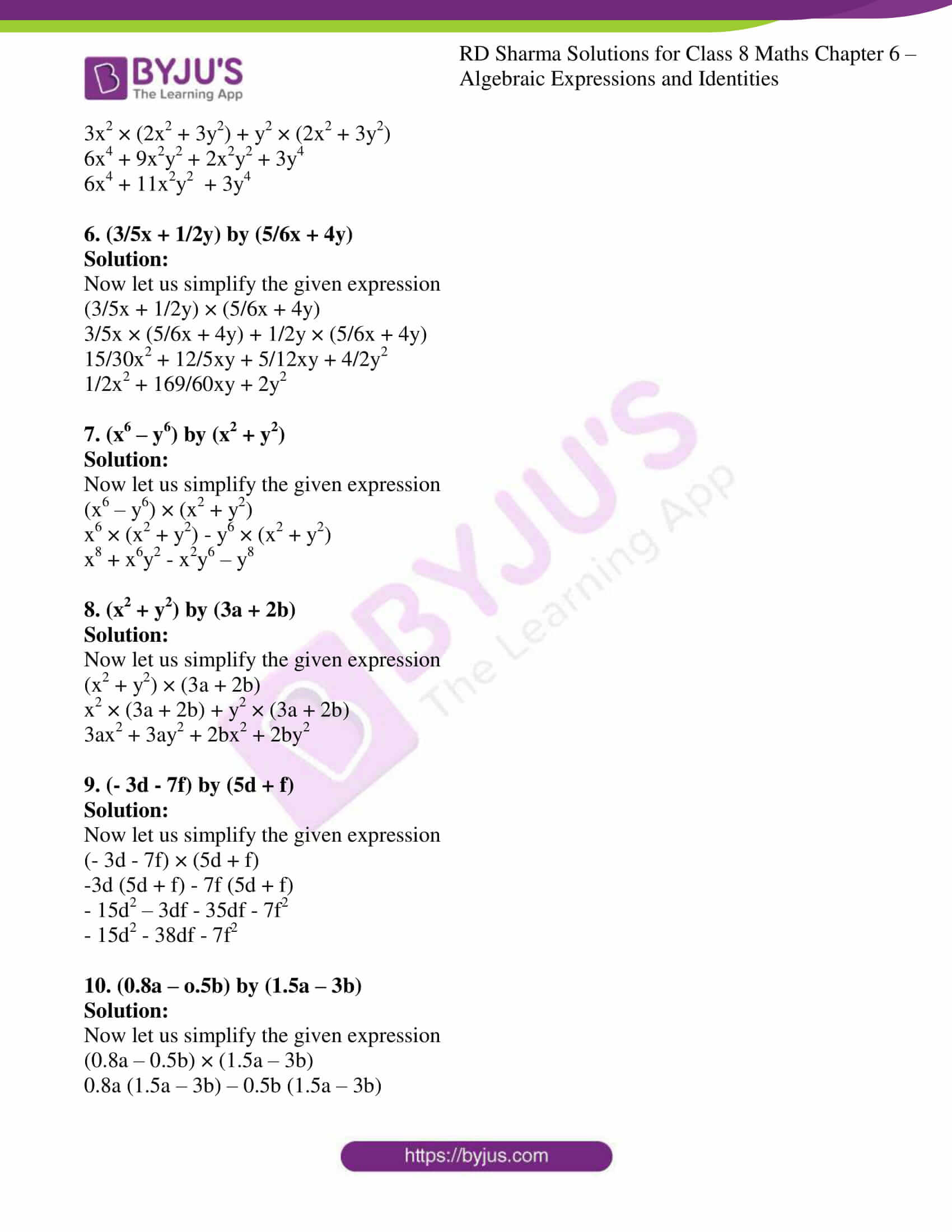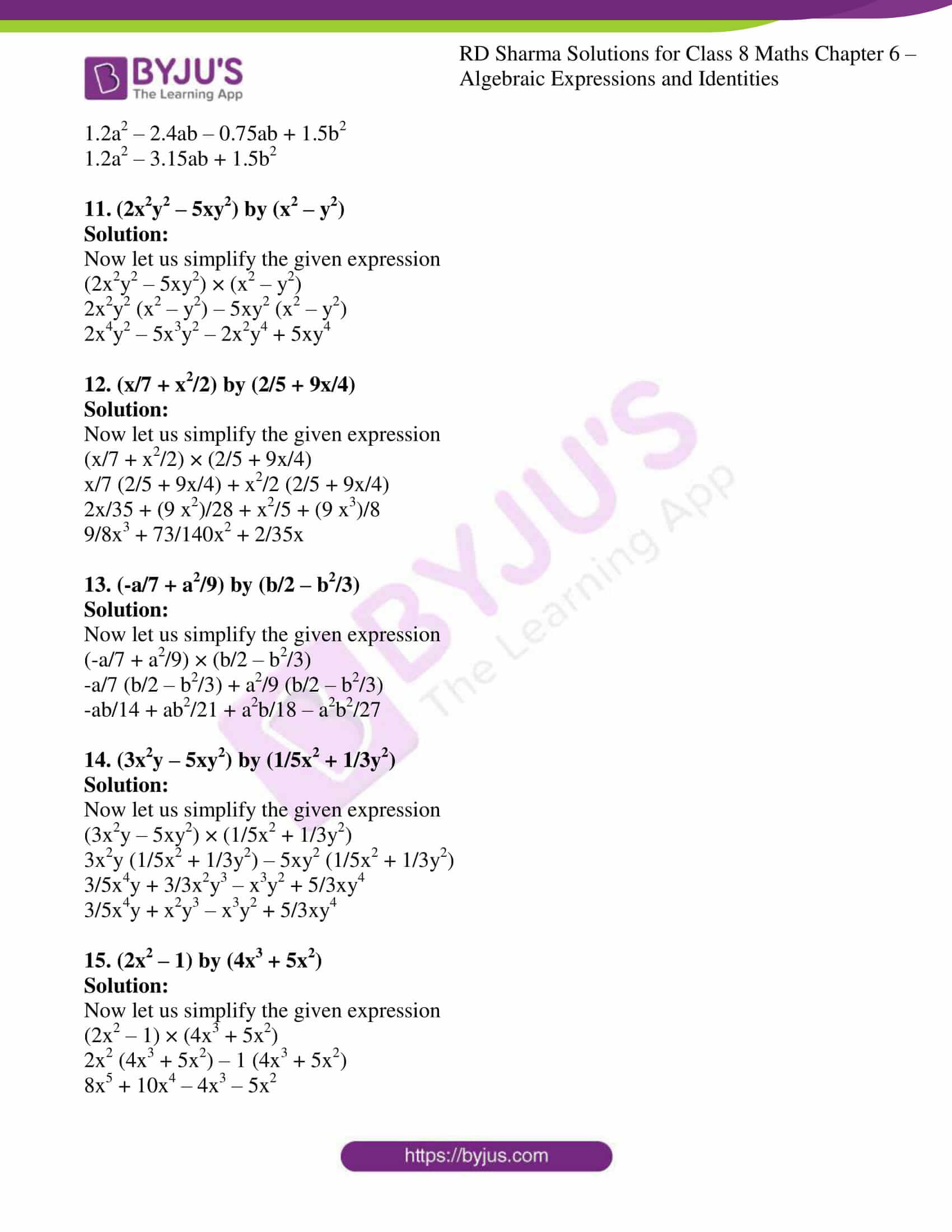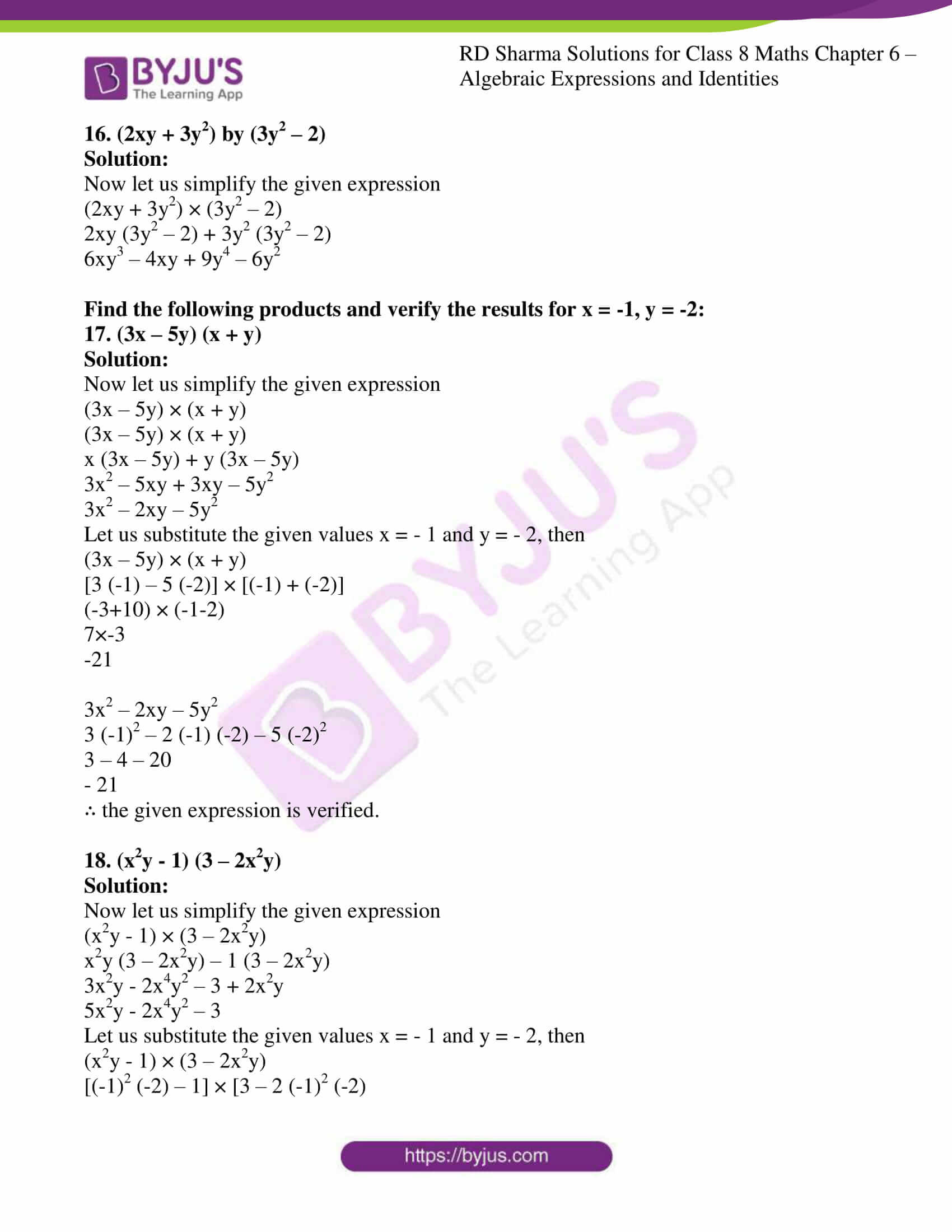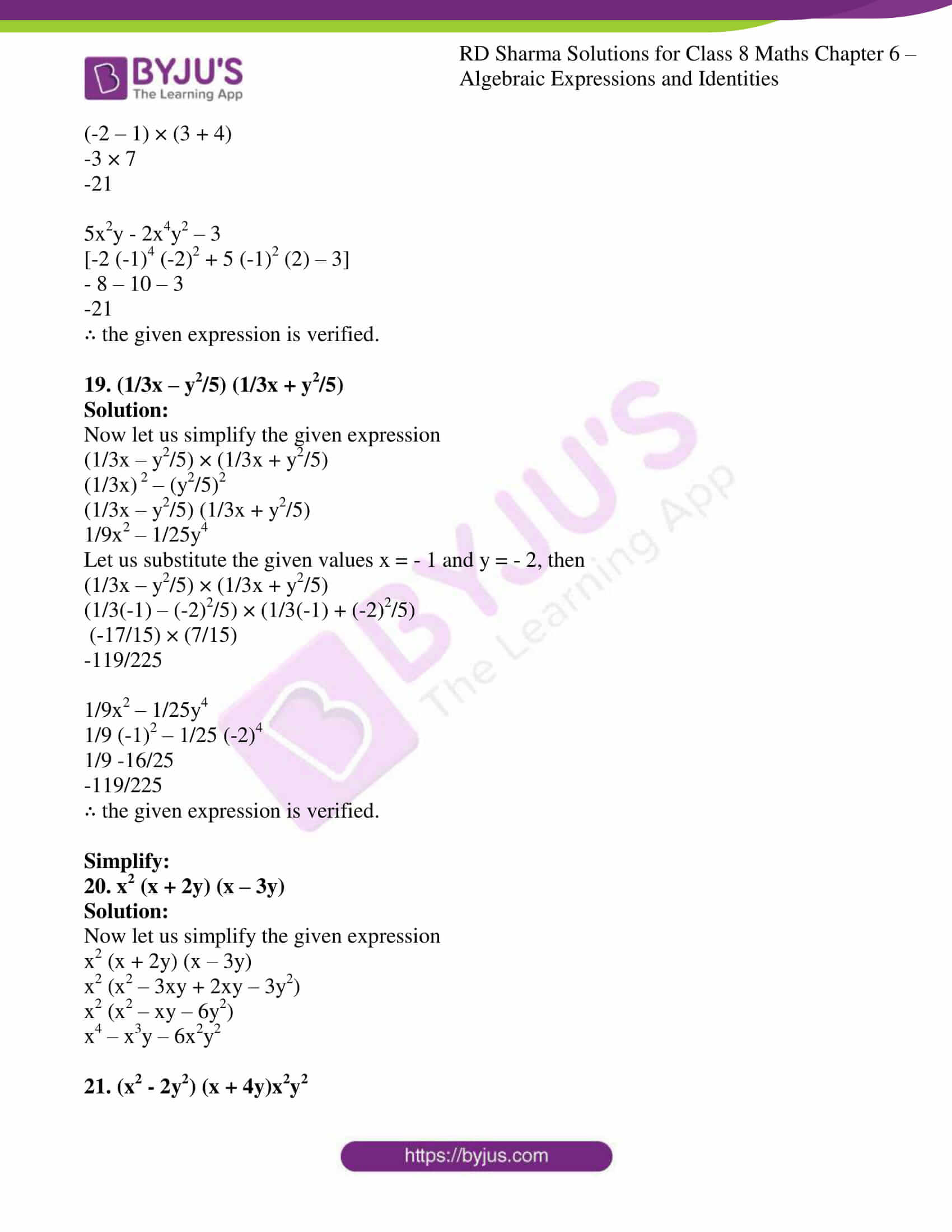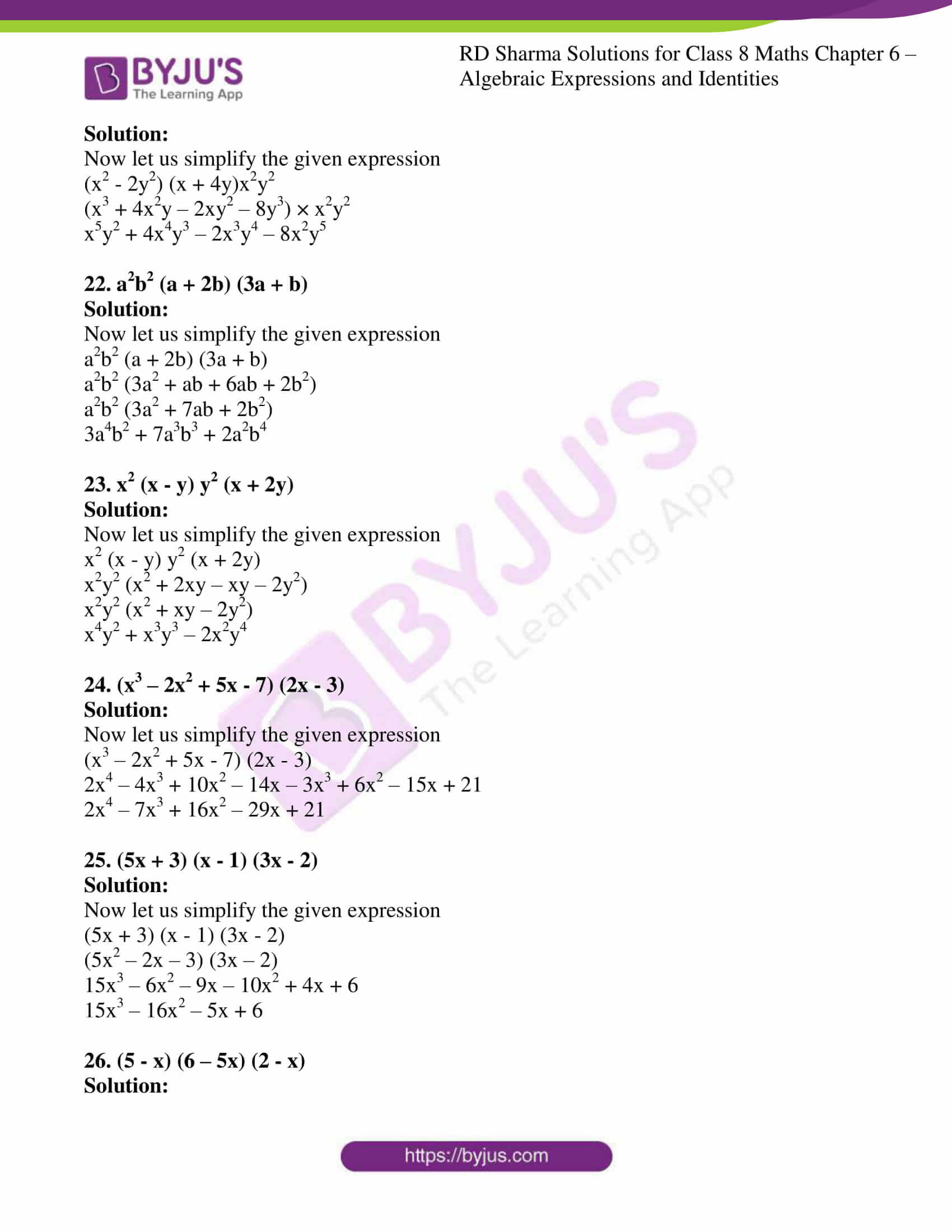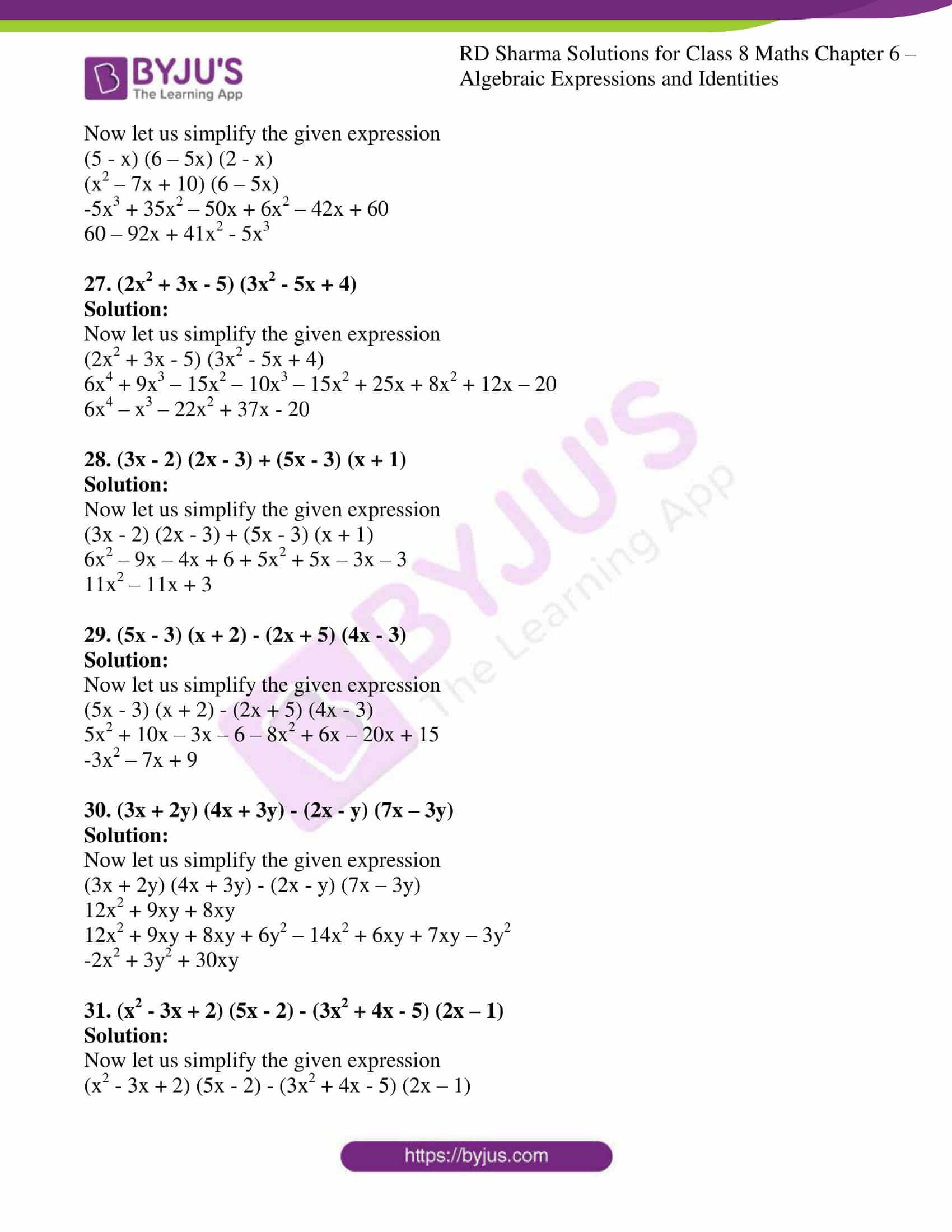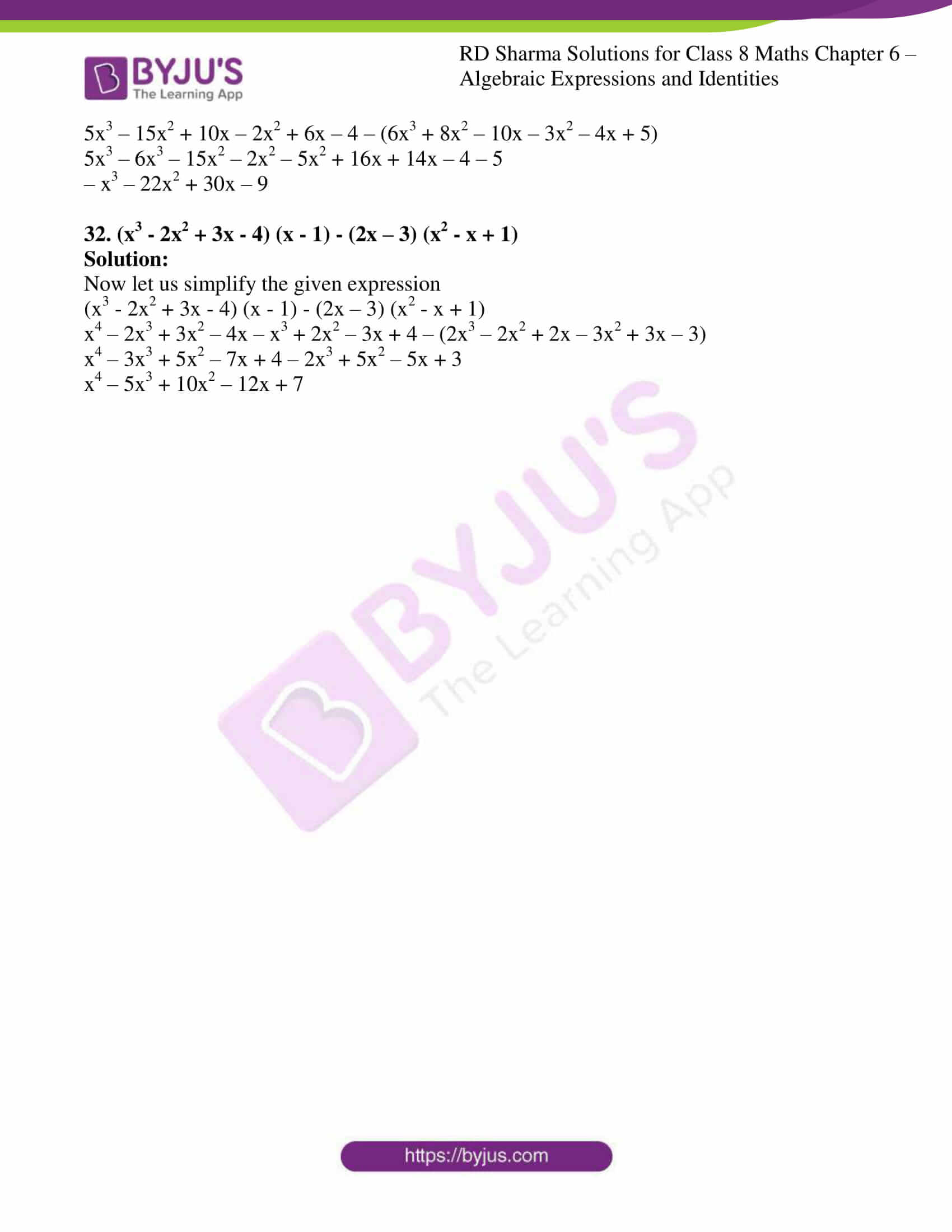### Access Answers to RD Sharma Solutions for Class 8 Maths Exercise 6.5 Chapter 6 Algebraic Expressions and Identities

#### EXERCISE 6.5 PAGE NO: 6.30

Multiply:

1. (5x + 3) by (7x + 2)

Solution:

Now let us simplify the given expression

(5x + 3) × (7x + 2)

5x (7x + 2) + 3 (7x + 2)

35x2 + 10x + 21x + 6

35x2 + 31x + 6

2. (2x + 8) by (x – 3)

Solution:

Now let us simplify the given expression

(2x + 8) × (x – 3)

2x (x – 3) + 8 (x – 3)

2x2 – 6x + 8x – 24

2x2 + 2x – 24

3. (7x + y) by (x + 5y)

Solution:

Now let us simplify the given expression

(7x + y) × (x + 5y)

7x (x + 5y) + y (x + 5y)

7x2 + 35xy + xy + 5y2

7x2 + 36xy + 5y2

4. (a – 1) by (0.1a2 + 3)

Solution:

Now let us simplify the given expression

(a – 1) × (0.1a2 + 3)

a (0.1a2 + 3) -1 (0.1a2 + 3)

0.1a3 + 3a – 0.1a2 – 3

0.1a3 – 0.1a2 + 3a – 3

5. (3x2 + y2) by (2x2 + 3y2)

Solution:

Now let us simplify the given expression

(3x2 + y2) × (2x2 + 3 y2)

3x2 × (2x2 + 3y2) + y2 × (2x2 + 3y2)

6x4 + 9x2y2 + 2x2y2 + 3y4

6x4 + 11x2y2 + 3y4

6. (3/5x + 1/2y) by (5/6x + 4y)

Solution:

Now let us simplify the given expression

(3/5x + 1/2y) × (5/6x + 4y)

3/5x × (5/6x + 4y) + 1/2y × (5/6x + 4y)

15/30x2 + 12/5xy + 5/12xy + 4/2y2

1/2x2 + 169/60xy + 2y2

7. (x6 – y6) by (x2 + y2)

Solution:

Now let us simplify the given expression

(x6 – y6) × (x2 + y2)

x6 × (x2 + y2) – y6 × (x2 + y2)

x8 + x6y2 – x2y6 – y8

8. (x2 + y2) by (3a + 2b)

Solution:

Now let us simplify the given expression

(x2 + y2) × (3a + 2b)

x2 × (3a + 2b) + y2 × (3a + 2b)

3ax2 + 3ay2 + 2bx2 + 2by2

9. (- 3d – 7f) by (5d + f)

Solution:

Now let us simplify the given expression

(- 3d – 7f) × (5d + f)

-3d (5d + f) – 7f (5d + f)

– 15d2 – 3df – 35df – 7f2

– 15d2 – 38df – 7f2

10. (0.8a – o.5b) by (1.5a – 3b)

Solution:

Now let us simplify the given expression

(0.8a – 0.5b) × (1.5a – 3b)

0.8a (1.5a – 3b) – 0.5b (1.5a – 3b)

1.2a2 – 2.4ab – 0.75ab + 1.5b2

1.2a2 – 3.15ab + 1.5b2

11. (2x2y2 – 5xy2) by (x2 – y2)

Solution:

Now let us simplify the given expression

(2x2y2 – 5xy2) × (x2 – y2)

2x2y2 (x2 – y2) – 5xy2 (x2 – y2)

2x4y2 – 5x3y2 – 2x2y4 + 5xy4

12. (x/7 + x2/2) by (2/5 + 9x/4)

Solution:

Now let us simplify the given expression

(x/7 + x2/2) × (2/5 + 9x/4)

x/7 (2/5 + 9x/4) + x2/2 (2/5 + 9x/4)

2x/35 + (9 x2)/28 + x2/5 + (9 x3)/8

9/8x3 + 73/140x2 + 2/35x

13. (-a/7 + a2/9) by (b/2 – b2/3)

Solution:

Now let us simplify the given expression

(-a/7 + a2/9) × (b/2 – b2/3)

-a/7 (b/2 – b2/3) + a2/9 (b/2 – b2/3)

-ab/14 + ab2/21 + a2b/18 – a2b2/27

14. (3x2y – 5xy2) by (1/5x2 + 1/3y2)

Solution:

Now let us simplify the given expression

(3x2y – 5xy2) × (1/5x2 + 1/3y2)

3x2y (1/5x2 + 1/3y2) – 5xy2 (1/5x2 + 1/3y2)

3/5x4y + 3/3x2y3 – x3y2 + 5/3xy4

3/5x4y + x2y3 – x3y2 + 5/3xy4

15. (2x2 – 1) by (4x3 + 5x2)

Solution:

Now let us simplify the given expression

(2x2 – 1) × (4x3 + 5x2)

2x2 (4x3 + 5x2) – 1 (4x3 + 5x2)

8x5 + 10x4 – 4x3 – 5x2

16. (2xy + 3y2) by (3y2 – 2)

Solution:

Now let us simplify the given expression

(2xy + 3y2) × (3y2 – 2)

2xy (3y2 – 2) + 3y2 (3y2 – 2)

6xy3 – 4xy + 9y4 – 6y2

Find the following products and verify the results for x = -1, y = -2:

17. (3x – 5y) (x + y)

Solution:

Now let us simplify the given expression

(3x – 5y) × (x + y)

(3x – 5y) × (x + y)

x (3x – 5y) + y (3x – 5y)

3x2 – 5xy + 3xy – 5y2

3x2 – 2xy – 5y2

Let us substitute the given values x = – 1 and y = – 2, then

(3x – 5y) × (x + y)

[3 (-1) – 5 (-2)] × [(-1) + (-2)]

(-3+10) × (-1-2)

7×-3

-21

3x2 – 2xy – 5y2

3 (-1)2 – 2 (-1) (-2) – 5 (-2)2

3 – 4 – 20

– 21

∴ the given expression is verified.

18. (x2y – 1) (3 – 2x2y)

Solution:

Now let us simplify the given expression

(x2y – 1) × (3 – 2x2y)

x2y (3 – 2x2y) – 1 (3 – 2x2y)

3x2y – 2x4y2 – 3 + 2x2y

5x2y – 2x4y2 – 3

Let us substitute the given values x = – 1 and y = – 2, then

(x2y – 1) × (3 – 2x2y)

[(-1)2 (-2) – 1] × [3 – 2 (-1)2 (-2)

(-2 – 1) × (3 + 4)

-3 × 7

-21

5x2y – 2x4y2 – 3

[-2 (-1)4 (-2)2 + 5 (-1)2 (2) – 3]

– 8 – 10 – 3

-21

∴ the given expression is verified.

19. (1/3x – y2/5) (1/3x + y2/5)

Solution:

Now let us simplify the given expression

(1/3x – y2/5) × (1/3x + y2/5)

(1/3x) 2 – (y2/5)2

(1/3x – y2/5) (1/3x + y2/5)

1/9x2 – 1/25y4

Let us substitute the given values x = – 1 and y = – 2, then

(1/3x – y2/5) × (1/3x + y2/5)

(1/3(-1) – (-2)2/5) × (1/3(-1) + (-2)2/5)

(-17/15) × (7/15)

-119/225

1/9x2 – 1/25y4

1/9 (-1)2 – 1/25 (-2)4

1/9 -16/25

-119/225

∴ the given expression is verified.

Simplify:

20. x2 (x + 2y) (x – 3y)

Solution:

Now let us simplify the given expression

x2 (x + 2y) (x – 3y)

x2 (x2 – 3xy + 2xy – 3y2)

x2 (x2 – xy – 6y2)

x4 – x3y – 6x2y2

21. (x2 – 2y2) (x + 4y)x2y2

Solution:

Now let us simplify the given expression

(x2 – 2y2) (x + 4y)x2y2

(x3 + 4x2y – 2xy2 – 8y3) × x2y2

x5y2 + 4x4y3 – 2x3y4 – 8x2y5

22. a2b2 (a + 2b) (3a + b)

Solution:

Now let us simplify the given expression

a2b2 (a + 2b) (3a + b)

a2b2 (3a2 + ab + 6ab + 2b2)

a2b2 (3a2 + 7ab + 2b2)

3a4b2 + 7a3b3 + 2a2b4

23. x2 (x – y) y2 (x + 2y)

Solution:

Now let us simplify the given expression

x2 (x – y) y2 (x + 2y)

x2y2 (x2 + 2xy – xy – 2y2)

x2y2 (x2 + xy – 2y2)

x4y2 + x3y3 – 2x2y4

24. (x3 – 2x2 + 5x – 7) (2x – 3)

Solution:

Now let us simplify the given expression

(x3 – 2x2 + 5x – 7) (2x – 3)

2x4 – 4x3 + 10x2 – 14x – 3x3 + 6x2 – 15x + 21

2x4 – 7x3 + 16x2 – 29x + 21

25. (5x + 3) (x – 1) (3x – 2)

Solution:

Now let us simplify the given expression

(5x + 3) (x – 1) (3x – 2)

(5x2 – 2x – 3) (3x – 2)

15x3 – 6x2 – 9x – 10x2 + 4x + 6

15x3 – 16x2 – 5x + 6

26. (5 – x) (6 – 5x) (2 – x)

Solution:

Now let us simplify the given expression

(5 – x) (6 – 5x) (2 – x)

(x2 – 7x + 10) (6 – 5x)

-5x3 + 35x2 – 50x + 6x2 – 42x + 60

60 – 92x + 41x2 – 5x3

27. (2x2 + 3x – 5) (3x2 – 5x + 4)

Solution:

Now let us simplify the given expression

(2x2 + 3x – 5) (3x2 – 5x + 4)

6x4 + 9x3 – 15x2 – 10x3 – 15x2 + 25x + 8x2 + 12x – 20

6x4 – x3 – 22x2 + 37x – 20

28. (3x – 2) (2x – 3) + (5x – 3) (x + 1)

Solution:

Now let us simplify the given expression

(3x – 2) (2x – 3) + (5x – 3) (x + 1)

6x2 – 9x – 4x + 6 + 5x2 + 5x – 3x – 3

11x2 – 11x + 3

29. (5x – 3) (x + 2) – (2x + 5) (4x – 3)

Solution:

Now let us simplify the given expression

(5x – 3) (x + 2) – (2x + 5) (4x – 3)

5x2 + 10x – 3x – 6 – 8x2 + 6x – 20x + 15

-3x2 – 7x + 9

30. (3x + 2y) (4x + 3y) – (2x – y) (7x – 3y)

Solution:

Now let us simplify the given expression

(3x + 2y) (4x + 3y) – (2x – y) (7x – 3y)

12x2 + 9xy + 8xy

12x2 + 9xy + 8xy + 6y2 – 14x2 + 6xy + 7xy – 3y2

-2x2 + 3y2 + 30xy

31. (x2 – 3x + 2) (5x – 2) – (3x2 + 4x – 5) (2x – 1)

Solution:

Now let us simplify the given expression

(x2 – 3x + 2) (5x – 2) – (3x2 + 4x – 5) (2x – 1)

5x3 – 15x2 + 10x – 2x2 + 6x – 4 – (6x3 + 8x2 – 10x – 3x2 – 4x + 5)

5x3 – 6x3 – 15x2 – 2x2 – 5x2 + 16x + 14x – 4 – 5

– x3 – 22x2 + 30x – 9

32. (x3 – 2x2 + 3x – 4) (x – 1) – (2x – 3) (x2 – x + 1)

Solution:

Now let us simplify the given expression

(x3 – 2x2 + 3x – 4) (x – 1) – (2x – 3) (x2 – x + 1)

x4 – 2x3 + 3x2 – 4x – x3 + 2x2 – 3x + 4 – (2x3 – 2x2 + 2x – 3x2 + 3x – 3)

x4 – 3x3 + 5x2 – 7x + 4 – 2x3 + 5x2 – 5x + 3

x4 – 5x3 + 10x2 – 12x + 7